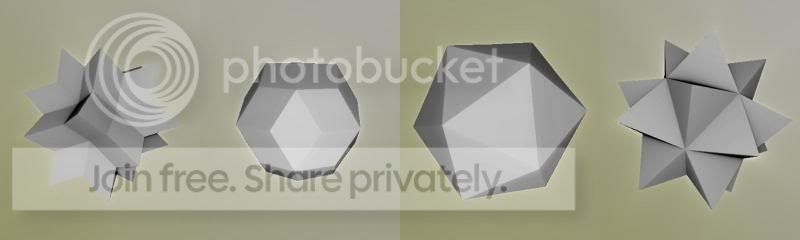# pouët.net

Go to bottom

category: code [glöplog]
Quote:
The Menger is an excellent base to create buildings, towers and the like.

oh really
Quote:
The Menger is an excellent base to create buildings, towers and the like.

oh really

:)
Code:`The Menger is an excellent base to create buildings, towers and the like.`

oh really
STOP IT NOW. Behave. ;)
*puts away manger joke and walks away*
Really!
Sorry, couldn't resist. :))
Fold and cut polyhedra:
Code:```float DE(vec3 z) { // Fold: //This is a folding set with dodecahedral symmetry z = abs(z); z-=2.0 * max(0.0, dot(z, n1)) * n1;//Thanks AndyAlias for the optimization z-=2.0 * max(0.0, dot(z, n2)) * n2; z = abs(z); z-=2.0 * max(0.0, dot(z, n1)) * n1; z-=2.0 * max(0.0, dot(z, n2)) * n2; z = abs(z); z-=2.0 * max(0.0, dot(z, n1)) * n1; z-=2.0 * max(0.0, dot(z, n2)) * n2; //Cut: //Distance to the plane going through vec3(Size,0.,0.) and which normal is plnormal (must be normalized) //You can also use curved and/or multiple cuts return dot(z-vec3(Size,0.,0.),plnormal); } ```
any screenshot ?
Thanks a lot for all your DE work knighty, i don't know how we could visualize all these fractals without you. (: And the first one in your link reminds surprisingly of a recent 4k intro too. ;)
I like the last examples , especially the subblue link.
Sorry for emphasize obvious facts.
nystep: ;o)

iq: About "cantor fracals", there is also this thread where I've given the method to get the distance estimate. I'm not surprised that it was already used in demoscene but it's a pitty such techniques are keps secret for so long time. That said I'm still wondering how the menger sponge was rendered in stargazer.

tigrou: It's cool you found the same technique as KIFS to render the Menger sponge. :)You can of course experiment with other folding planes.

Maybe this is a little bit out of subject but It's related to it and I thought you may find this of some interrest: using foldings to generate triangle group tilings.
here is what I did so far:
evaldraw script
fragmentarium scripts

here is where that idea came from:
"kleinian drops" boxplorer scripts. There is an hyperbolic tesselation hiding in one of the examples. ;)
video by marius.
geometry:
Code:``` Octahedron: float octahedron(float3 p, float size) { p=abs(p); return (p.x+p.y+p.z-size)/3; } Rounded Cubes: float rcube(float3 p,float3 box,float rad ) { return length(max(abs(p)-box+rad,0.0))-rad; } Hexagon: float hexagonX(float3 p, float x,float y ) { p=abs(p); return max(p.x-y,max(p.y+p.z*.5,p.z)-x); } Rings: float ring(float3 p,float r,float r2,float c) { return max(abs(length(p.xz)-r)-r2,abs(p.y)-c); } ```
the hexagon is just on x-plane, also the dividing is not correct, but smaller! (for 4k-usage) ;)
Does anyone have a copy of zeno.pdf that they can upload? I can't seem to find it anywhere and the links posted earlier are both dead.
I also want to mention that the "Hexagonal Prism - unsigned" isn't really symmetric.
IQ's dist functions

Code:``` q.x+q.y*0.57735 ```

should maybe be (coeffs are sin/cos of 60°)

Code:``` q.x*0.866025 + q.y*0.5 ```
I did some RM stuff a while back and stumbled onto the scale repeater thing, I'm sure u guys have done it but just in case:

I'm not sure how it works - since it was a pure accident for me - I was trying to do something else.

this was the start of the math fumbling

and ended up with this

never had so much fun before :P
las:

cos(60) / sin(60) = 0.57735
ooops, you are right, i missed one multiplication!!!!
correct code:

Code:``` float udHexPrism( vec3 p, vec2 h ) { float q = abs(p); return max(q.z-h.y,max(q.x+q.y*0.57735,q.y*1.154700538)-h.x); } ```

(q is a vec3, of course)
0.57735 = 1/sqrt(3), 1.1547=2/sqrt(3)Code:``` #ifdef GL_ES precision highp float; #endif uniform vec2 resolution; float udHexPrism( vec2 p, float h ) { vec2 q = abs(p); return max(q.x+q.y*0.57735,q.y*1.1547)-h; } void main(void) { vec2 p = -1.0 + 2.0 * gl_FragCoord.xy / resolution.xy; p.x *= 1.3333; float d1 = udHexPrism( p, 1.0 ); float d2 = length(p) - 1.0; float f = 0.0; if( d1<0.0 ) f=1.0; float g = 0.0; if( d2<0.0 ) g=1.0; vec3 col = vec3(f,g,0.0); gl_FragColor = vec4(col,1.0); } ```

i'll fix my website
Here is a comparison of both versions:

"inner" hexagon (yours)
"outer" hexagon (what I proposed)

There are good reasons for both versions - I really prefer the "outer" version for e.g. you have a "circle packing" and use the outer version on it -> it will be space filling.

And the "outer" version has another plus - you can easily express it as dot product (well depends whether it's really a plus - but I like dot products):
Code:``` float udHexPrism( vec2 p, float h ) { vec2 q = abs(p); //return max(q.x*0.866025 + q.y*0.5, q.y)-h; // can also be expressed as dot prod. return max(dot(q, vec2(0.866025, 0.5)), q.y)-h; } ```

btw. there are still some people expecting an e-mail ;D
i like inners in general (hex, tris, quads, ...) cause then the meaning of the "radious" sort of makes sense, which comes helpful for me when i have to pack or combine geometry, as i know exactly the bounds of my objects and therefore i know when things will touch, etc. but i suppose is a matter or taste and depends no the application. also, i noticed that in fact this gives negative distances in the inside, although i didn't check if they give the correct distance (the images look promising). can anybody check???

as for the email, i stopped the project i wanted to work on. perhaps i'll resume it in 2013...!